# Transistor Oscillator : Circuit, Working & Its Applications

Generally, the oscillator is an electronic device used to change the DC energy into AC energy with a high frequency where the frequency ranges from Hz to some MHz.  An oscillator does not need any exterior signal source, like an amplifier. Generally, oscillators are available in two types sinusoidal & non-sinusoidal. The oscillations generated by sinusoidal oscillators are sine waves formed at stable frequency & amplitude whereas the oscillations generated by non-sinusoidal are complex waveforms like Triangular, Square-wave, and Sawtooth. So this article discusses an overview of a transistor as an oscillator or transistor oscillator – working with applications.

## Define Transistor Oscillator

When a transistor acts as an oscillator with proper positive feedback then it is known as a transistor oscillator. This oscillator generates undamped oscillations continuously for any desired frequency if tank & feedback circuits are connected to it properly.

### Transistor Oscillator Circuit Diagram

The circuit diagram of the transistor oscillator is shown below. By using this circuit, we can simply explain how to utilize a transistor as an oscillator. This circuit is separated into three parts like the following.

#### Tank Circuit

The tank circuit generates oscillations that are changed with the transistor & generates amplified output within the collector side.

#### Amplifier Circuit

This circuit is used to amplify the tiny sinusoidal oscillations available within the base-emitter circuit & output is produced in the amplified form.

#### Feedback Circuit

The feedback circuit is a very significant section in this circuit because, for an amplifier, it requires some energy to amplify at the tank circuit. So, the energy of the collector circuit is fed back to the base circuit using the Mutual induction phenomenon. By using this circuit, the energy is fed back from the output to the input.

#### Working of Transistor as an Oscillator

In the above transistor oscillator circuit, the transistor is used as a CE (common emitter) circuit where the emitter is common to both the base & collector terminals. Between the emitter and base input terminals, a tank circuit is connected. In the tank circuit, the inductor & capacitor are parallelly connected to generate oscillations within the circuit.

Because of the voltage & charge oscillations within the tank circuit, the flow of current at the base terminal fluctuates, so the forward biasing of the base current periodically changes then the collector current also changes periodically.

LC oscillations are sinusoidal in nature so both the base and collector currents sinusoidally vary. As shown in the diagram, if the current at the collector terminal sinusoidally changes then the output voltage attained can be simply written as Ic RL. This output is considered a sinusoidal output.

Once we draw a graph in-between time and output voltage then the curve will be sinusoidal. To get oscillations continuously within the tank circuit,  we require some energy. But in this circuit, no dc source or battery is available.

So we connected L1 & L2 inductors within the collector & base circuits using a soft iron rod. So this rod will connect the L2 inductor to L1 inductor because of its mutual induction, A part of the energy within the collector circuit will be connected to the base side of the circuit. Thus, the oscillation within the tank circuit is sustained & amplified continuously.

#### Oscillation Conditions

The transistor oscillator circuit must follow the following

• The phase shift of the loop should be 0 & 360 degrees.
• The loop gain must be >1.
• If a sinusoidal signal is a preferred output, then a loop gain > 1 will quickly cause the o/p to saturate at both waveform peaks & generating unacceptable distortion.
• If the gain of the amplifier is >100, then it will cause the oscillator to limit both the waveform peaks. To meet the above conditions, the oscillator circuit should include some type of amplifier, as well as a part of its output, which should be fed back to the input. To conquer the losses within the input circuit, we utilize the feedback circuit. If the amplifier’s gain is <1, then the oscillator circuit will not oscillate and if it is > 1, then the circuit will oscillate and generate distorted signals.

### Types of Transistor Oscillator

There are different kinds of oscillators available but each oscillator has the same function. So they generate continuous undamped output. But, they change in supplying energy to the oscillatory or tank circuit to meet the frequency ranges as well as losses over which they are utilized.

Transistor oscillators which use LC circuits as their oscillatory or tank circuits are extremely popular for producing high-frequency outputs. The different types of transistor oscillators are discussed below.

#### Hartley Oscillator

The Hartley oscillator is one kind of electronic oscillator that is used to determine the oscillation frequency through a tuned circuit. The main feature of this oscillator is that the tuned circuit includes a single capacitor connected in parallel through two inductors in series & the feedback signal required for oscillation is obtained from the two inductors’ center connection. Hartley oscillator is appropriate for oscillations in the RF range up to 30MHz. To know more about this oscillator click here – Hartley oscillator.

#### Crystal Oscillator

Transistor crystal oscillator is applicable in different areas of electronics as well as radio. These types of oscillators play a key role in providing a cheap CLK signal to use in logic or digital circuit. In other examples, this oscillator may be used for providing a constant and precise RF signal source. So these oscillators are frequently used by radio amateurs or radio hams within radio transmitter circuits, wherever they can be most effective. To know more about this oscillator click here – crystal oscillator.

#### Colpitt’s Oscillator

The Colpitts oscillator is quite opposite to Hartley Oscillator except the inductors & capacitors are replaced with each other within the tank circuit. The main benefit of this kind of oscillator is that by less mutual & self-inductance in the tank circuit, the oscillator’s frequency stability is improved. This oscillator generates very high frequencies based on sinusoidal signals. These oscillators have high-frequency stability and they can withstand low and high temperatures. To know more about this oscillator click here – Colpitts oscillator

#### Wien Bridge Oscillator

Wien bridge oscillator is an audio frequency oscillator that is frequently used due to its significant features. This type of oscillator is free from fluctuations as well as the ambient temperature of the circuit The main benefit of this kind of oscillator is that the frequency is changed from 10Hz to 1MHz range. So this oscillator circuit gives good stability of frequency. To know more about this oscillator click here – Wien bridge oscillator.

#### Phase Shift Oscillator

RC phase shift oscillator is one kind of oscillator wherever a simple RC network is used to provide the necessary phase shift toward the feedback signal. Similar to Hartley & Colpitts oscillator, this oscillator uses an LC network to provide the required positive feedback. This oscillator has outstanding frequency stability and it generates pure sine waves over an extensive range of loads. To know more about this oscillator click here – RC phase shift oscillator

### Frequency ranges of different transistor oscillators are:

• wien bridge (1Hz to 1MHz),
• phase shift oscillator (1Hz to 10MHz),
• Hartley oscillator (10kHz to 100MHz),
• Colpitts (10kHz to 100MHz) &
• negative resistance oscillator >100MHz

#### Transistor Oscillator using Resonant Circuit

A transistor oscillator using a resonant circuit including an inductor & a capacitor within a series will generate frequency oscillations. If an inductor is doubled & capacitor will be changed to 4C, then the frequency is given by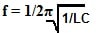The above frequency expression is used for the frequency of LC oscillations within a series LC circuit. After that, finding the two frequencies like f1 & f2 ratio, and substituting the changes within the inductance & the capacitance values, the ‘f2’ frequency can be found in terms of ‘f1’.

The two frequencies (f1&f2) ratio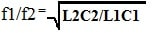Here ‘L’ is doubled & ‘C’ is changed to 4C

Substitute these values in the above equation, then we can get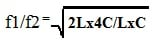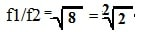If we find the ‘f2’ frequency in terms of the ‘f1’ frequency then we can get the following equation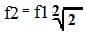### Applications

The applications of a transistor as an oscillator include the following.

• A transistor oscillator is used to generate constant undamped oscillations for any desired frequency if oscillatory & feedback circuits are connected properly to it.
• Wien bridge oscillator is highly used in audio testing, power amplifiers distortion testing, and also used for the AC bridge excitation.